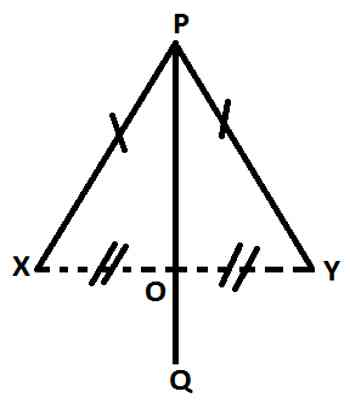Theorems on Locus of a Point

Locus is a curve or other figure formed by all the points satisfying a particular equation of the relation between coordinates, or by a point, line, or surface moving according to mathematically defined conditions. A path consisting of a set of points whose location is governed by some definite rule of rules is called locus.

Theorems on Locus of a Point which is Equidistant from Two Fixed Points

The locus of a point which is equidistant from two fixed points is the perpendicular bisector of the line segment joining the two fixed points.

Given,

Let X and Y be two given fixed points. PQ is the path traced out by the moving point P such that each point on it is equidistant from X and Y. Therefore, PX = PY.

To prove: PQ is the perpendicular bisector of the line segment XY.

Construction: Join X to Y. Let PQ cut XY at O.Proof

From △PXO and △PYO,

PX and PY (Given)

XO = YO (Since, every point of PQ is equidistant from X and Y, and O is a point on PQ.)

PO = PO (Common side.)

Therefore, by the SSS criterion of congruency △PXO ≅ △PYO.

Now ∠POX = ∠POY (since, corresponding parts of congruent triangles are congruent.)

Again ∠POX + ∠POY = 180° (Since, XOY is a straight line.

Therefore, ∠POX = ∠POY = 180°/2 = 90°

Also, PQ bisects XY (Since, XO = YO)

Therefore, PQ ⊥ XY and PQ bisects XY, i.e., PQ is the perpendicular bisector of XY (Proved)

Information Source: